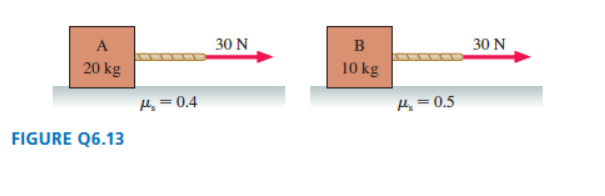# Problem: Boxes A and B in FIGURE Q6.13 both remain at test. Is the friction force on A larger than, smaller than, or equal to the friction force on B? Explain.

###### FREE Expert Solution

Since both box A and box B remain at rest, the force equivalent to the applied force acts in the opposite direction.

84% (379 ratings)###### Problem Details

Boxes A and B in FIGURE Q6.13 both remain at test. Is the friction force on A larger than, smaller than, or equal to the friction force on B? Explain.